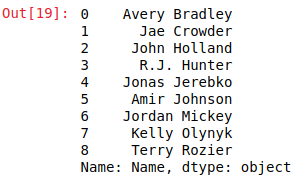## Python | Pandas Dataframe/Series.head() method

Python is a great language for doing data analysis, primarily because of the fantastic ecosystem of data-centric Python packages. Pandas is one of those packages and makes importing and analyzing data much easier.

Pandas `head()` method is used to return top n (5 by default) rows of a data frame or series.

Parameters:
n: integer value, number of rows to be returned

Return type: Dataframe with top n rows

In the following examples, the data frame used contains data of some NBA players. The image of data frame before any operations is attached below.Example #1:

In this example, top 5 rows of data frame are returned and stored in a new variable. No parameter is passed to .head() method since by default it is 5.

 `# importing pandas module` `import` `pandas as pd` ` ` `# making data frame` `data ``=` `pd.read_csv(``"https://media.geeksforgeeks.org/wp-content/uploads/nba.csv"``)` ` ` `# calling head() method ` `# storing in new variable` `data_top ``=` `data.head()` ` ` `# display` `data_top`

Output:
As shown in the output image, it can be seen that the index of returned rows is ranging from 0 to 4. Hence, top 5 rows were returned.Example #2: Calling on Series with n parameter()

In this example, the .head() method is called on series with custom input of n parameter to return top 9 rows of the series.

 `# importing pandas module` `import` `pandas as pd` ` ` `# making data frame` `data ``=` `pd.read_csv(``"https://media.geeksforgeeks.org/wp-content/uploads/nba.csv"``)` ` ` `# number of rows to return` `n ``=` `9` ` ` `# creating series` `series ``=` `data[``"Name"``]` ` ` `# returning top n rows` `top ``=` `series.head(n ``=` `n)` ` ` `# display` `top`

Output:
As shown in the output image, top 9 rows ranging from 0 to 8th index position were returned.Last Updated on March 1, 2022 by admin

## Seaborn.barplot() method in PythonSeaborn.barplot() method in Python

Seaborn.barplot() method in Python Seaborn is a Python data visualization library based on Matplotlib. It provides

## Action Chains in Selenium PythonAction Chains in Selenium Python

Action Chains in Selenium Python Selenium’s Python Module is built to perform automated testing with

## Normal Equation in Linear RegressionNormal Equation in Linear Regression

ML | Normal Equation in Linear Regression Normal Equation is an analytical approach to Linear Regression

## IntegerField – Django ModelsIntegerField – Django Models

IntegerField – Django Models IntegerField is a integer number represented in Python by a int instance. This field

## numpy.cov() functionnumpy.cov() function

Python | numpy.cov() function Covariance provides the a measure of strength of correlation between two

## Classifying data using Support Vector Machines(SVMs) in PythonClassifying data using Support Vector Machines(SVMs) in Python

Classifying data using Support Vector Machines(SVMs) in Python Introduction to SVMs: In machine learning, support

## Analysis of Different Methods to find Prime Number in PythonAnalysis of Different Methods to find Prime Number in Python

Analysis of Different Methods to find Prime Number in Python If you participate in competitive

## Python program to input a comma separated stringPython program to input a comma separated string

Python program to input a comma separated string Given an input string that is comma-separated